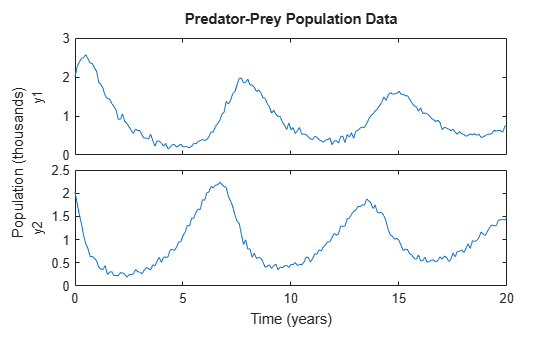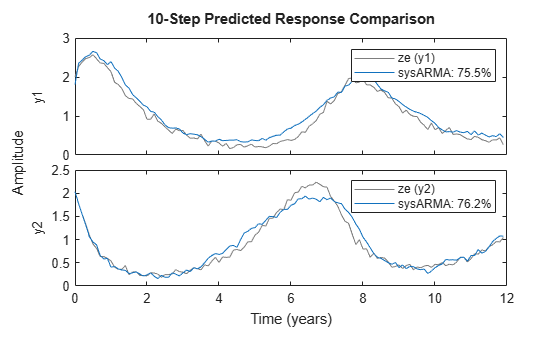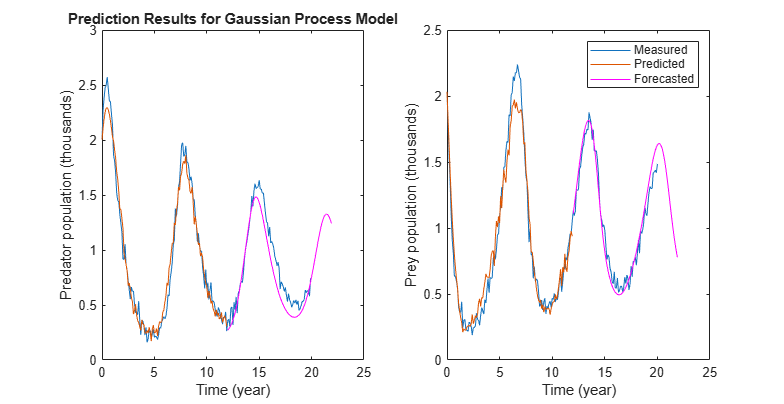# Forecast Multivariate Time Series

This example shows how to perform multivariate time series forecasting of data measured from predator and prey populations in a prey crowding scenario. The predator-prey population-change dynamics are modeled using linear and nonlinear time series models. Forecasting performance of these models is compared.

### Data Description

The data is a bivariate time series consisting of 1-predator 1-prey populations (in thousands) collected 10 times a year for 20 years. For more information about the data, see Three Ecological Population Systems: MATLAB and C MEX-File Modeling of Time-Series.

```load PredPreyCrowdingData z = iddata(y,[],0.1,'TimeUnit','years','Tstart',0);```

`z` is an `iddata` object containing two output signals, `y1` and `y2`, which refer to the predator and prey populations, respectively. The `OutputData` property of `z` contains the population data as a 201-by-2 matrix, such that `z.OutputData(:,1)` is the predator population and `z.OutputData(:,2)` is the prey population.

Plot the data.

```plot(z) title('Predator-Prey Population Data') ylabel('Population (thousands)')```The data exhibits a decline in predator population due to crowding.

Use the first half as estimation data for identifying time series models.

`ze = z(1:120);`

Use the remaining data to search for model orders, and to validate the forecasting results.

`zv = z(121:end);`

### Estimate a Linear Model

Model the time series as a linear, autoregressive process. Linear models can be created in polynomial form or state-space form using commands such as `ar` (for scalar time series only), `arx`, `armax`, `n4sid` and `ssest`. Since the linear models do not capture the data offsets (non-zero conditional mean), first detrend the data.

```[zed, Tze] = detrend(ze, 0); [zvd, Tzv] = detrend(zv, 0);```

Identification requires specification of model orders. For polynomial models, you can find suitable orders using the `arxstruc` command. Since `arxstruc `works only on single-output models, perform the model order search separately for each output.

```na_list = (1:10)'; V1 = arxstruc(zed(:,1,:),zvd(:,1,:),na_list); na1 = selstruc(V1,0); V2 = arxstruc(zed(:,2,:),zvd(:,2,:),na_list); na2 = selstruc(V2,0);```

The `arxstruc` command suggests autoregressive models of orders 7 and 8, respectively.

Use these model orders to estimate a multi-variance ARMA model where the cross terms have been chosen arbitrarily.

```na = [na1 na1-1; na2-1 na2]; nc = [na1; na2]; sysARMA = armax(zed,[na nc])```
```sysARMA = Discrete-time ARMA model: Model for output "y1": A(z)y_1(t) = - A_i(z)y_i(t) + C(z)e_1(t) A(z) = 1 - 0.885 z^-1 - 0.1493 z^-2 + 0.8089 z^-3 - 0.2661 z^-4 - 0.9487 z^-5 + 0.8719 z^-6 - 0.2896 z^-7 A_2(z) = 0.3433 z^-1 - 0.2802 z^-2 - 0.04949 z^-3 + 0.1018 z^-4 - 0.02683 z^-5 - 0.2416 z^-6 C(z) = 1 - 0.4534 z^-1 - 0.4127 z^-2 + 0.7874 z^-3 + 0.298 z^-4 - 0.8684 z^-5 + 0.6106 z^-6 + 0.3616 z^-7 Model for output "y2": A(z)y_2(t) = - A_i(z)y_i(t) + C(z)e_2(t) A(z) = 1 - 0.5826 z^-1 - 0.4688 z^-2 - 0.5949 z^-3 - 0.0547 z^-4 + 0.5062 z^-5 + 0.4024 z^-6 - 0.01544 z^-7 - 0.1766 z^-8 A_1(z) = 0.2386 z^-1 + 0.1564 z^-2 - 0.2249 z^-3 - 0.2638 z^-4 - 0.1019 z^-5 - 0.07821 z^-6 + 0.2982 z^-7 C(z) = 1 - 0.1717 z^-1 - 0.09877 z^-2 - 0.5289 z^-3 - 0.24 z^-4 + 0.06555 z^-5 + 0.2217 z^-6 - 0.05765 z^-7 - 0.1824 z^-8 Sample time: 0.1 years Parameterization: Polynomial orders: na=[7 6;7 8] nc=[7;8] Number of free coefficients: 43 Use "polydata", "getpvec", "getcov" for parameters and their uncertainties. Status: Estimated using ARMAX on time domain data "zed". Fit to estimation data: [89.85;90.97]% (prediction focus) FPE: 3.814e-05, MSE: 0.007533 ```

Compute a 10-step-ahead (1 year) predicted output to validate the model over the time span of the estimation data. Since the data was detrended for estimation, you need to specify those offsets for meaningful predictions.

```predOpt = predictOptions('OutputOffset',Tze.OutputOffset'); yhat1 = predict(sysARMA,ze,10, predOpt);```

The `predict` command predicts the response over the time span of measured data and is a tool for validating the quality of an estimated model. The response at time `t` is computed using measured values at times t = 0, ..., t-10.

Plot the predicted response and the measured data.

```plot(ze,yhat1) title('10-step predicted response compared to measured data')```Note, the generation of predicted response and plotting it with the measured data, can be automated using the `compare` command.

```compareOpt = compareOptions('OutputOffset',Tze.OutputOffset'); compare(ze,sysARMA,10,compareOpt)```The plot generated using `compare` also shows the normalized root mean square (NRMSE) measure of goodness of fit in percent form.

After validating the data, forecast the output of the model `sysARMA` 100 steps (10 years) beyond the estimation data, and calculate output standard deviations.

```forecastOpt = forecastOptions('OutputOffset',Tze.OutputOffset'); [yf1,x01,sysf1,ysd1] = forecast(sysARMA, ze, 100, forecastOpt);```

`yf1` is the forecasted response, returned as an `iddata` object. `yf1.OutputData` contains the forecasted values.

`sysf1` is a system similar to `sysARMA` but is in state-space form. Simulation of `sysf1` using the `sim` command, with initial conditions, `x01`, reproduces the forecasted response, `yf1`.

`ysd1` is the matrix of standard deviations. It measures the uncertainty is forecasting owing to the effect of additive disturbances in the data (as measured by `sysARMA.NoiseVariance`), parameter uncertainty (as reported by `getcov(sysARMA)`) and uncertainties associated with the process of mapping past data to the initial conditions required for forecasting (see `data2state`).

Plot the measured, predicted, and forecasted output for model `sysARMA`.

```t = yf1.SamplingInstants; te = ze.SamplingInstants; t0 = z.SamplingInstants; subplot(1,2,1); plot(t0,z.y(:,1),... te,yhat1.y(:,1),... t,yf1.y(:,1),'m',... t,yf1.y(:,1)+ysd1(:,1),'k--', ... t,yf1.y(:,1)-ysd1(:,1), 'k--') xlabel('Time (year)'); ylabel('Predator population, in thousands'); subplot(1,2,2); plot(t0,z.y(:,2),... te,yhat1.y(:,2),... t,yf1.y(:,2),'m',... t,yf1.y(:,2)+ysd1(:,2),'k--', ... t,yf1.y(:,2)-ysd1(:,2),'k--') % Make the figure larger. fig = gcf; p = fig.Position; fig.Position = [p(1),p(2)-p(4)*0.2,p(3)*1.4,p(4)*1.2]; xlabel('Time (year)'); ylabel('Prey population, in thousands'); legend({'Measured','Predicted','Forecasted','Forecast Uncertainty (1 sd)'},... 'Location','best')```The plots show that forecasting using a linear ARMA model (with added handling of offsets) worked somewhat and the results showed high uncertainty compared to the actual populations over the 12-20 years time span. This indicates that the population change dynamics might be nonlinear.

### Estimate a Nonlinear Black-Box Model

Fit a nonlinear black-box model to the estimation data. You do not require prior knowledge about the equations governing the estimation data. A linear-in-regressor form of Nonlinear ARX model will be estimated.

Create a nonlinear ARX model with 2 outputs and no inputs.

`sysNLARX = idnlarx([1 1;1 1],[],'Ts',0.1,'TimeUnit','years');`

`sysNLARX` is a first order nonlinear ARX model that uses no nonlinear function; it predicts the model response using a weighted sum of its first-order regressors.

`getreg(sysNLARX)`
```Regressors: For output 1: y1(t-1) y2(t-1) For output 2: y1(t-1) y2(t-1) ```

To introduce a nonlinearity function, add polynomial regressors to the model.

Create regressors up to power 2, and include cross terms (products of standard regressors listed above). Add those regressors to the model as custom regressors.

```R = polyreg(sysNLARX,'MaxPower',2,'CrossTerm','on'); sysNLARX.CustomRegressors = R; getreg(sysNLARX)```
```Regressors: For output 1: y1(t-1) y2(t-1) y1(t-1).^2 y1(t-1).*y2(t-1) y2(t-1).^2 For output 2: y1(t-1) y2(t-1) y1(t-1).^2 y1(t-1).*y2(t-1) y2(t-1).^2 ```

Estimate the coefficients (the regressor weightings and the offset) of the model using estimation data, `ze`.

`sysNLARX = nlarx(ze,sysNLARX)`
```sysNLARX = Nonlinear ARX model with 2 outputs and 0 inputs Inputs: Outputs: y1, y2 Standard regressors corresponding to the orders: na = [1 1; 1 1] nb = [] nk = [] Custom regressors: For output 1: y1(t-1).^2 y1(t-1).*y2(t-1) y2(t-1).^2 For output 2: y1(t-1).^2 y1(t-1).*y2(t-1) y2(t-1).^2 Nonlinear regressors: For output 1: none For output 2: none Model output is linear in their regressors. Sample time: 0.1 years Status: Estimated using NLARX on time domain data "ze". Fit to estimation data: [88.34;88.91]% (prediction focus) FPE: 3.265e-05, MSE: 0.01048 ```

Compute a 10-step-ahead predicted output to validate the model.

`yhat2 = predict(sysNLARX,ze,10);`

Forecast the output of the model 100 steps beyond the estimation data.

`yf2 = forecast(sysNLARX,ze,100);`

The standard deviations of the forecasted response are not computed for nonlinear ARX models. This data is unavailable because the parameter covariance information is not computed during estimation of these models.

Plot the measured, predicted, and forecasted outputs.

```t = yf2.SamplingInstants; subplot(1,2,1); plot(t0,z.y(:,1),... te,yhat2.y(:,1),... t,yf2.y(:,1),'m') xlabel('Time (year)'); ylabel('Predator population (thousands)'); subplot(1,2,2); plot(t0,z.y(:,2),... te,yhat2.y(:,2),... t,yf2.y(:,2),'m') legend('Measured','Predicted','Forecasted') xlabel('Time (year)'); ylabel('Prey population (thousands)');```The plots show that forecasting using a nonlinear ARX model gave better forecasting results than using a linear model. Nonlinear black-box modeling did not require prior knowledge about the equations governing the data.

Note that to reduce the number of regressors, you can pick an optimal subset of (transformed) variables using principal component analysis (see `pca`) or feature selection (see `sequentialfs`) in the Statistics and Machine Learning Toolbox™.

If you have prior knowledge of the system dynamics, you can fit the estimation data using a nonlinear grey-box model.

### Estimate a Nonlinear Grey-Box Model

Knowledge about the nature of the dynamics can help improve the model quality and thus the forecasting accuracy. For the predator-prey dynamics, the changes in the predator (`y1`) and prey (`y2`) population can be represented as:

`${\underset{}{\overset{˙}{y}}}_{1}\left(t\right)={p}_{1}*{y}_{1}\left(t\right)+{p}_{2}*{y}_{2}\left(t\right)*{y}_{1}\left(t\right)$`

`${\underset{}{\overset{˙}{y}}}_{2}\left(t\right)={p}_{3}*{y}_{2}\left(t\right)-{p}_{4}*{y}_{1}\left(t\right)*{y}_{2}\left(t\right)-{p}_{5}*{y}_{2}\left(t{\right)}^{2}$`

For more information about the equations, see Three Ecological Population Systems: MATLAB and C MEX-File Modeling of Time-Series.

Construct a nonlinear grey-box model based on these equations.

Specify a file describing the model structure for the predator-prey system. The file specifies the state derivatives and model outputs as a function of time, states, inputs, and model parameters. The two outputs (predator and prey populations) are chosen as states to derive a nonlinear state-space description of the dynamics.

`FileName = 'predprey2_m';`

Specify the model orders (number of outputs, inputs, and states)

`Order = [2 0 2];`

Specify the initial values for the parameters ${p}_{1}$, ${p}_{2}$, ${p}_{3}$, ${p}_{4}$, and ${p}_{5}$, and indicate that all parameters are to be estimated. Note that the requirement to specify initial guesses for parameters did not exist when estimating the black box models `sysARMA` and `sysNLARX`.

```Parameters = struct('Name',{'Survival factor, predators' 'Death factor, predators' ... 'Survival factor, preys' 'Death factor, preys' ... 'Crowding factor, preys'}, ... 'Unit',{'1/year' '1/year' '1/year' '1/year' '1/year'}, ... 'Value',{-1.1 0.9 1.1 0.9 0.2}, ... 'Minimum',{-Inf -Inf -Inf -Inf -Inf}, ... 'Maximum',{Inf Inf Inf Inf Inf}, ... 'Fixed',{false false false false false});```

Similarly, specify the initial states of the model, and indicate that both initial states are to be estimated.

```InitialStates = struct('Name',{'Predator population' 'Prey population'}, ... 'Unit',{'Size (thousands)' 'Size (thousands)'}, ... 'Value',{1.8 1.8}, ... 'Minimum', {0 0}, ... 'Maximum',{Inf Inf}, ... 'Fixed',{false false});```

Specify the model as a continuous-time system.

`Ts = 0;`

Create a nonlinear grey-box model with specified structure, parameters, and states.

`sysGrey = idnlgrey(FileName,Order,Parameters,InitialStates,Ts,'TimeUnit','years');`

Estimate the model parameters.

```sysGrey = nlgreyest(ze,sysGrey); present(sysGrey)```
``` sysGrey = Continuous-time nonlinear grey-box model defined by 'predprey2_m' (MATLAB file): dx/dt = F(t, x(t), p1, ..., p5) y(t) = H(t, x(t), p1, ..., p5) + e(t) with 0 input(s), 2 state(s), 2 output(s), and 5 free parameter(s) (out of 5). States: Initial value x(1) Predator population(t) [Size (thou..] xinit@exp1 2.01325 (estimated) in [0, Inf] x(2) Prey population(t) [Size (thou..] xinit@exp1 1.99687 (estimated) in [0, Inf] Outputs: y(1) y1(t) y(2) y2(t) Parameters: Value Standard Deviation p1 Survival factor, predators [1/year] -0.995895 0.0125269 (estimated) in [-Inf, Inf] p2 Death factor, predators [1/year] 1.00441 0.0129368 (estimated) in [-Inf, Inf] p3 Survival factor, preys [1/year] 1.01234 0.0135413 (estimated) in [-Inf, Inf] p4 Death factor, preys [1/year] 1.01909 0.0121026 (estimated) in [-Inf, Inf] p5 Crowding factor, preys [1/year] 0.103244 0.0039285 (estimated) in [-Inf, Inf] Status: Termination condition: Change in cost was less than the specified tolerance.. Number of iterations: 6, Number of function evaluations: 7 Estimated using Solver: ode45; Search: lsqnonlin on time domain data "ze". Fit to estimation data: [91.21;92.07]% FPE: 8.613e-06, MSE: 0.005713 More information in model's "Report" property. ```

Compute a 10-step-ahead predicted output to validate the model.

`yhat3 = predict(sysGrey,ze,10);`

Forecast the output of the model 100 steps beyond the estimation data, and calculate output standard deviations.

`[yf3,x03,sysf3,ysd3] = forecast(sysGrey,ze,100);`

Plot the measured, predicted, and forecasted outputs.

```t = yf3.SamplingInstants; subplot(1,2,1); plot(t0,z.y(:,1),... te,yhat3.y(:,1),... t,yf3.y(:,1),'m',... t,yf3.y(:,1)+ysd3(:,1),'k--', ... t,yf3.y(:,1)-ysd3(:,1),'k--') xlabel('Time (year)'); ylabel('Predator population (thousands)'); subplot(1,2,2); plot(t0,z.y(:,2),... te,yhat3.y(:,2),... t,yf3.y(:,2),'m',... t,yf3.y(:,2)+ysd3(:,2),'k--', ... t,yf3.y(:,2)-ysd3(:,2),'k--') legend('Measured','Predicted','Forecasted','Forecast uncertainty (1 sd)') xlabel('Time (years)'); ylabel('Prey population (thousands)');```The plots show that forecasting using a nonlinear grey-box model gave good forecasting results and low forecasting output uncertainty.

### Compare Forecasting Performance

Compare the forecasted response obtained from the identified models, `sysARMA`, `sysNLARX`, and `sysGrey`. The first two are discrete-time models and `sysGrey` is a continuous-time model.

```clf plot(z,yf1,yf2,yf3) legend({'Measured','Linear ARMA','Nonlinear AR','Nonlinear Grey-Box'}) title('Forecasted Responses')```Forecasting with a nonlinear ARX model gave better results than forecasting with a linear model. Inclusion of the knowledge of the dynamics in the nonlinear grey-box model further improved the reliability of the model and therefore the forecasting accuracy.

Note that the equations used in grey-box modeling are closely related to the polynomial regressors used by the Nonlinear ARX model. If you approximate the derivatives in the governing equations by first-order differences, you will get equations similar to:

`${y}_{1}\left(t\right)={s}_{1}*{y}_{1}\left(t-1\right)+{s}_{2}*{y}_{1}\left(t-1\right)*{y}_{2}\left(t-1\right)$`

`${y}_{2}\left(t\right)={s}_{3}*{y}_{2}\left(t-1\right)-{s}_{4}*{y}_{1}\left(t-1\right)*{y}_{2}\left(t-1\right)-{s}_{5}*{y}_{2}\left(t-1{\right)}^{2}$`

Where, ${s}_{1},...,{s}_{5}$ are some parameters related to the original parameters ${p}_{1},...,{p}_{5}$ and to the sample time used for differencing. These equations suggest that only 2 regressors are needed for the first output (y1(t-1) and *y1(t-1)*y2(t-1)) and 3 for the second output when constructing the Nonlinear ARX model. Even in absence of such prior knowledge, linear-in-regressor model structures employing polynomial regressors remain a popular choice in practice.

Forecast the values using the nonlinear grey-box model over 200 years.

`[yf4,~,~,ysd4] = forecast(sysGrey, ze, 2000);`

Plot the latter part of the data (showing 1 sd uncertainty)

```t = yf4.SamplingInstants; N = 700:2000; subplot(1,2,1); plot(t(N), yf4.y(N,1), 'm',... t(N), yf4.y(N,1)+ysd4(N,1), 'k--', ... t(N), yf4.y(N,1)-ysd4(N,1), 'k--') xlabel('Time (year)'); ylabel('Predator population (thousands)'); ax = gca; ax.YLim = [0.8 1]; ax.XLim = [82 212]; subplot(1,2,2); plot(t(N),yf4.y(N,2),'m',... t(N),yf4.y(N,2)+ysd4(N,2),'k--', ... t(N),yf4.y(N,2)-ysd4(N,2),'k--') legend('Forecasted population','Forecast uncertainty (1 sd)') xlabel('Time (years)'); ylabel('Prey population (thousands)'); ax = gca; ax.YLim = [0.9 1.1]; ax.XLim = [82 212];```The plot show that the predatory population is forecasted to reach a steady-state of approximately 890 and the prey population is forecasted to reach 990.

## SupportGet trial now